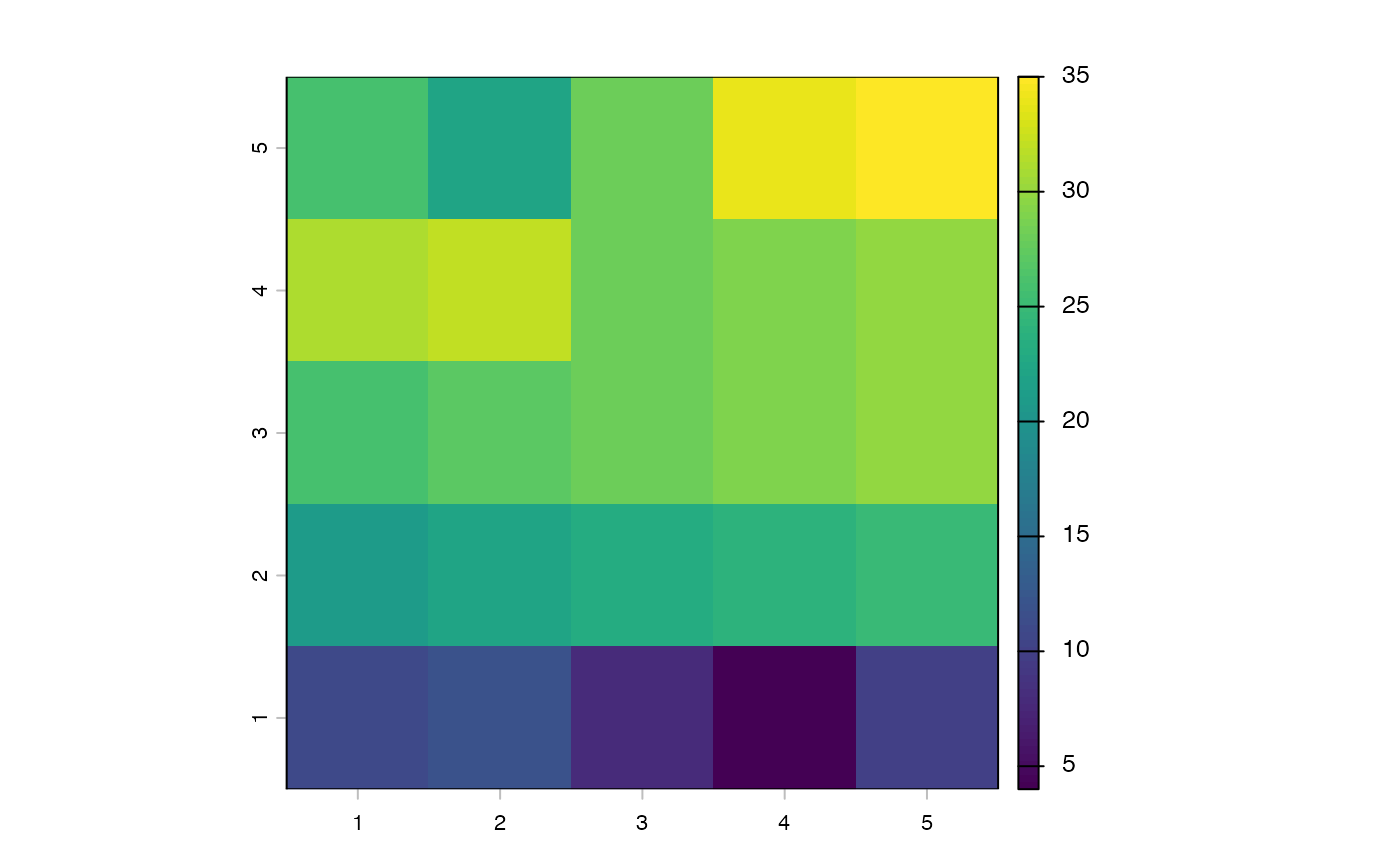Samples deepest depth values from a SpatVector data frame and generates a SpatRaster.

bottomRaster(rawPointData)

## Arguments

rawPointData

A SpatVector object from which bottom variables will be sampled. See Details for more about format.

## Value

A SpatRaster designed to approximate sea bottom measurements for modeling species' distributions and/or niches.

## Details

rawPointData is a SpatVector object that contains measurements of a single environmental variable (e.g. salinity, temperature, etc.) with x, y, and z coordinates. The measurements in the data.frame should be organized so that each column is a depth slice, increasing in depth from left to right. The function was designed around the oceanographic data shapefiles supplied by the World Ocean Atlas (https://www.ncei.noaa.gov/access/world-ocean-atlas-2018/). The function selects the "deepest" (rightmost) measurement at each x, y coordinate pair that contains data. These measurements are then rasterized at the resolution and extent of the x,y coordinates, under the assumption that x and y intervals are equal and represent the center of a cell.

## Examples


library(terra)

# Create point grid
coords <- data.frame(x = rep(seq(1:5), times = 5),
y = unlist(lapply(1:5, FUN = function(x) {
rep(x, times = 5)})))

# Create data and add NAs to simulate uneven bottom depths
dd <- data.frame(SURFACE = 1:25,
d5M = 6:30,
d10M = 11:35,
d25M = 16:40)
dd$d25M[c(1:5, 18:25)] <- NA dd$d10M[c(3:5, 21:23)] <- NA
dd\$d5M[c(4, 22)] <- NA

dd[,c("x","y")] <- coords

# Create SpatialPointsDataFrame
sp <- vect(dd, geom = c("x", "y"))

# Here's the function
result <- bottomRaster(rawPointData = sp)
plot(result)